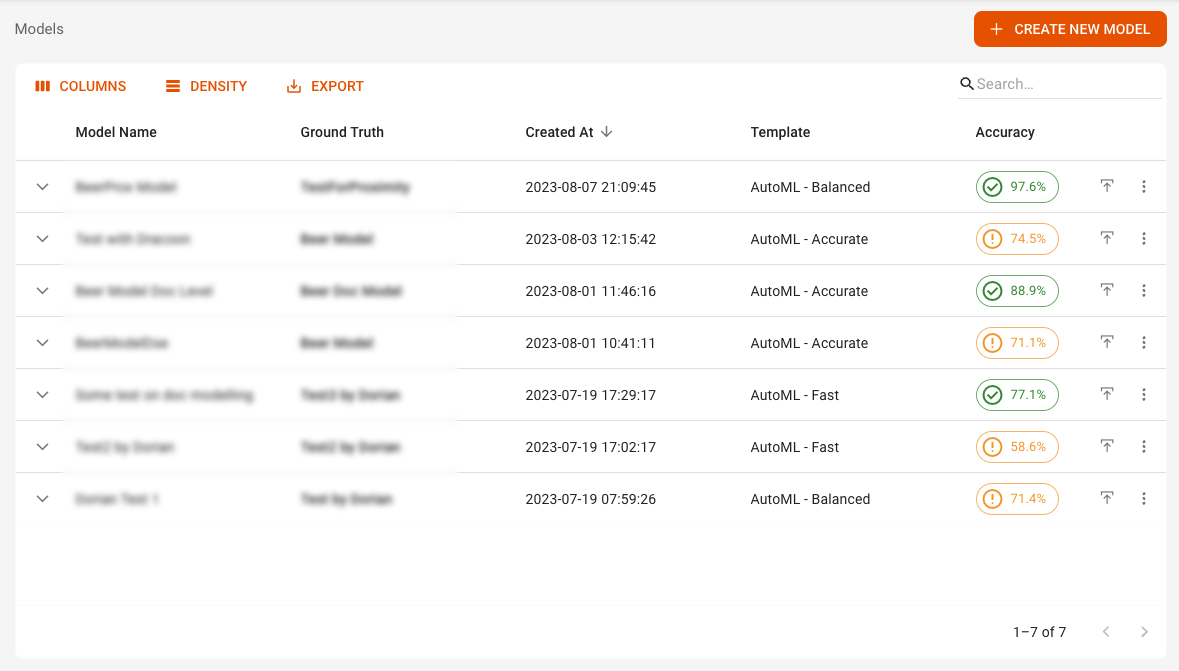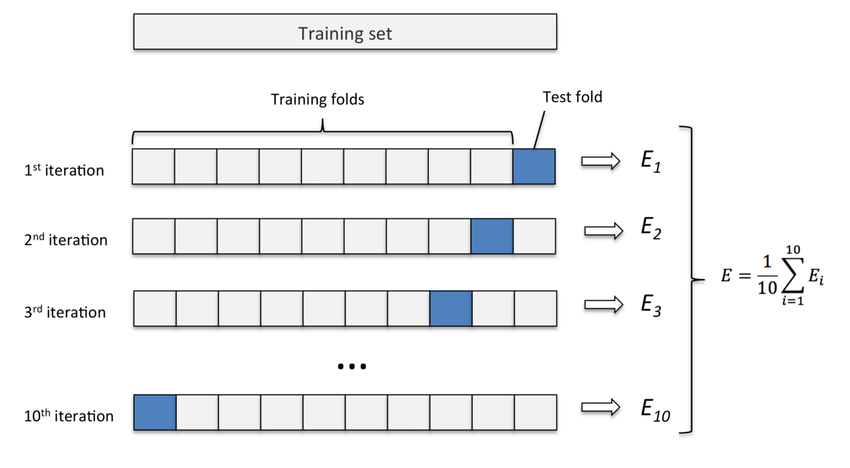# Models Overview#

Profiles: Model Creator, Data Scientist

After creating a ground truth based upon at least one candidate set, the second step within AI Studio is to create a model.

Reference: If you are looking for step-by-step instructions on how to build a sentence-level or document-level model in AI Studio, including creating ground truths, see the following:

## Introduction#

The Model is the component that performs text classification and consists of two main components:

• Ground Truth

• Machine Learning Workflow

Models are built off of Ground Truths, where the Model learns its behavior from the text extracts and labels in a ground truth set.

The Models Overview tab (Step 2 of AI Studio) has three primary screens:

• Models Overview screen, which allows you to create, duplicate, re-train, validate, delete, and publish models.

• Create New Model, which allows you to configure a new model by giving it a name and description and selecting the ground truth and template the model will use.

• Validation screen, which allows you to view the model’s accuracy, precision, and recall. You can also view correct and incorrect predictions. The validation screen also allows you to publish a model.

## Models Overview Screen#

After completing Step 1 of AI Studio, you’ll find yourself on the Models Overview screen, as shown in the screenshot below:Here you will see a list of models that are sortable using the Columns and Density icons. You can also click the dropdown arrow to the left of the model name to view additional information, including the date it was last trained.

For larger projects, you can also search for a specific model by using the Search bar.

Note: The Accuracy of the model is shown in the last column after the training process is finished.

### Actions#

From the Models overview screen, you can perform the following actions:

• Publish a model by clicking the publish icon next to the accuracy score.

• You can also click the three dots icon next to each model to perform the following actions, as shown in the screenshot below:• Duplicate: Copy the config of a model.

• Re-Train: Re-train a model based on the latest ground truth.

• Validate: Switch to the validation screen.

• Delete: Delete an unpublished model.

## Validation Screen#

In the Validation section you can see the performance of your model tested on the ground truth. The model gets tested only on unseen data, due to the k-fold validation approach. (See the section later on this page for more information on k-fold validation.)

In addition to common performance metrics and the confusion matrix, there is a detailed view of text extracts that have been classified correctly or incorrectly, as shown in the example screenshot below:### Understanding the Terminology#

AI Models are evaluated using the Accuracy, Precision, and Recall metrics, which are standard metrics for evaluating the performance of a classification model. They are defined as follows:

• Accuracy: How often a model is correct. It is calculated by dividing the number of correct predictions by the total number of predictions.

$accuracy = \frac{TP + TN}{TP + TN + FP + FN}$
• Precision: How often a model is correct when it predicts a positive result. It is calculated by dividing the number of correct positive predictions by the total number of correct positive predictions plus false positive predictions.

$precision = \frac{TP}{TP + FP}$
• Recall: How often a model predicts a positive result when the result is actually positive. It is calculated by dividing the number of correct positive predictions by the total number of correct positive predictions plus false positive predictions.

$Recall = \frac{TP}{TP + FN}$

Reference: To learn more, see the following external guide: Accuracy vs. precision vs. recall in machine learning: what’s the difference?.

### Correcting Poor Performance#

In situations where the performance of the trained model falls below expectations, the following steps can be taken to improve results:

• Try another template.

• Check which examples got miss classified and add more similar examples.

• Add more examples to the ground truth.

• Try to build a successful proximity rule model first and use the bulk labeling feature to expand your ground truth set.

## K-Fold Cross Validation#

K-fold cross-validation is primarily used in applied machine learning to estimate the performance of a machine learning model on unseen data.

It is a re-sampling procedure to evaluate machine learning models in limited data.

The k-fold step splits the data set into k different subsets and iterates over them using one of them as the test set and the remaining k-1 elements as the training set.

The figure below shows an example for k = 10:### Parameters#

• k: how many pieces the data set gets split into.

• output_path: file path in which the output of the k-fold validation gets stored.

• output_field: field in which the predicitons are going to be stored in.

• classifier_params: parameter of a lib.nlp classifier to be used during the k-fold validation

Note: classifier and label fields exist for inheritance and are not used.

### Example#

{
"step": "classifier",
"type": "kfold",
"k": 5,
"output_path": "./output.json",
"output_field": "prediction",
"label_field": "class",
"classifier": "none",
"classifier_params":   {
"explanation_field": "explanation",
"input_fields": [
"normalized_extract"
],
"label_field": "label",
"model_kwargs": {
"probability": true
},
"model_type": "SVC",
"output_field": "prediction",
"step": "classifier",
"type": "sklearn"
}
}


### Output#

It outputs the success rate for each group of the k folds.

Additionally, it lists the overall metrics of the output.

Note: In the case of multiclass classification, the precision, recall, and f1-score are macro averaged.

****** KFOLD VALIDATION OUTPUT *****
Group 0:{'successful predicted': 26, 'total': 27, 'ratio': 0.9629629629629629}
Group 1:{'successful predicted': 27, 'total': 27, 'ratio': 1.0}
Group 2:{'successful predicted': 27, 'total': 27, 'ratio': 1.0}
Group 3:{'successful predicted': 25, 'total': 27, 'ratio': 0.9259259259259259}
Group 4:{'successful predicted': 27, 'total': 27, 'ratio': 1.0}
Report saved into: ./output.json
**********************************

****** OVERALL METRICS *****
metrics: {'metrics': {'accuracy': 97.2, 'precision': 97.39999999999999, 'recall': 97.2, 'f1-score': 97.2}, 'confusion_matrix': {'labels': ['cat', 'not_cat'], 'values': [17, 0, 1, 18]}}
Report stored at: ./kfold_validation.json
**********************************